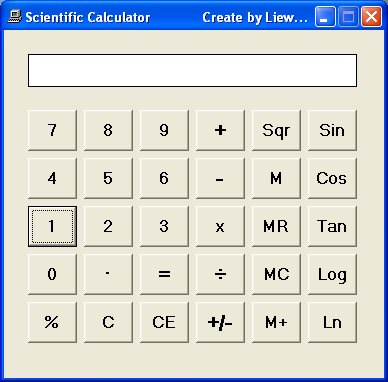# Scientific Calculator

This is a calculator that resembles a typical scientific calculator , albeit a simpler version. In our version, we have only included the trigonometric functions and the logarithmic functions. The reason of creating a simpler version of the calculator is to help users to learn the programming concepts in a gradual manner and not to confuse them especially those who are learning to program in Visual Basic. To design the interface, we just to need to modify the interface of the basic calculator that we have created earlier using Visual Basic 6.  In this calculator, we have added five more buttons, they are Sin, Cos, Tan, Log and Ln.  The common trigonometric functions in Visual Basic 6 are Sin, Cos, Tan and Atn as explained below:

1. Sin is the function that computes the value of sine of an angle in radian.
2. Cos is the function that computes the value of cosine of an angle in radian.
3. Tan is the function that computes the value of tangent of an angle in radian.
4. Atn is the function that computes the value of arc tangent of an angle in radian.

Log computes the value of logarithm to base 10 whilst Ln computes the value of natural logarithm.

An angle in degree has to be converted to radian before it can be calculated by the above trigonometric functions. From high school mathematics, we know that π radian is equivalent to 180°; which means 1 radian is equivalent to π divided by 180. Therefore, in order to convert an angle x from degree to radian, we have to multiply x by (π/180). However, there is a small problem because it is rather difficult to obtain the precise value of π, fortunately, there is a way to do it in VB. First of all, we know that an arc tangent of 1 will return the value of 45° which is π/4 radian. So, to obtain the value of π, just multiply the arc tangent of 1 with 4. Now, we are ready to enter the code for all the above additional buttons.

For the Sin button, enter the following code:

```Private Sub CmdSin_Click()

sinx = Round(Sin(displayValue * 4 * Atn(1) / 180), 4)
panel.Caption = sinx

End Sub
```
* The Round function is to correct the output to certain number of decimal places.

For the Cos button, enter the following code:

```Private Sub CmdCos_Click()

cosx = Round(Cos(displayValue * 4 * Atn(1) / 180), 4)
panel.Caption = cosx

End Sub
```

For the Tan button, enter the following code:

```Private Sub CmdTan_Click()

tanx = Round(Tan(displayValue * 4 * Atn(1) / 180), 4)
panel.Caption = tanx

End Sub
```

For the Ln button, enter the following code:

```Private Sub CmdLn_Click()
panel.Caption = Log(displayValue)
End Sub
```

and for the Log button, enter the following code:

```Private Sub CmdLog_Click()
panel.Caption = Log(displayValue) / Log(10)
End Sub
```

The interface is shown below: